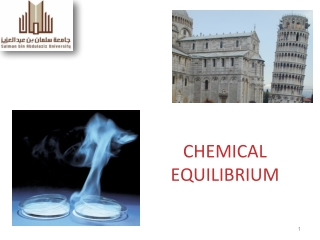DownloadDownload PresentationCHEMICAL EQUILIBRIUM

# CHEMICAL EQUILIBRIUM

Download Presentation## CHEMICAL EQUILIBRIUM

- - - - - - - - - - - - - - - - - - - - - - - - - - - E N D - - - - - - - - - - - - - - - - - - - - - - - - - - -
##### Presentation Transcript

1. CHEMICAL EQUILIBRIUM

2. Chemical Equilibrium • Chemical Reactions Types; • What is equilibrium? • Expressions for equilibrium constants, Kc; • Calculating Kc using equilibrium concentrations; • Calculating equilibrium concentrations using initial concentration and Kc value; • Relationship between Kc and Kp; • Factors that affect equilibrium; • Le Chatelier’s Principle • Application on Le Chatelier’s Principle

3. Chemical Reactions Chemical Reactions divided into two categories: • Reactants consumed by the end of the reaction, and product concentration reaches maximum (reaction occurred in one direction). • Both reactants and products are present  at certain concentrations by the end of the reaction (Reaction occurred in two directions).

4. Reactions in two directions They have both the forward and reverse reactions. We reperesnt the chemical equation with a double arrow:

5. Chemical Equilibrium Equilibrium: no physical change with time (no observable changes as time goes by). Chemical equilibrium is achieved when: 1.) The rates of the forward and reverse reactions are equal. 2.) The concentrations of the reactants and products remain constant

6. Equilibrium • There are two types of equilibrium: Physical and Chemical. • Physical Equilibrium • H20 (l) ↔ H20 (g) • Chemical Equilibrium • N2O4 (g) ↔ 2NO2 (g)

7. The Concept of Equilibrium Chemical equilibrium occurs when a reaction and its reverse reaction proceed at the same rate.

8. The Equilibrium Constant Forward reaction: Rate law Reverse reaction: Rate Law

9. The Equilibrium Constant At equilibrium Rearranging gives:

10. The Equilibrium Constant The ratio of the rate constants is a constant (as long as T is constant). The expression becomes

11. Law of Mass Action Law of Mass Action- For a reversible reaction at equilibrium and constant temperature, rate of any chemical reaction is proportional to the concentrations of product and reactants, each concentration raised to a power equal to the stoichiometry coefficient . The Equilibrium Constant (K)- the ratio of the equilibrium concentrations of products to the equilibrium concentrations of reactants each raised to the power of its stoichiometric coefficient.

12. The Equilibrium Constant To generalize, the reaction: Has the equilibrium expression:

13. SAMPLE EXERCISE : Writing Equilibrium-Constant Expressions • Write the equilibrium expression for Kc for the following reactions: Solution Strategy: Using the law of mass action, the product concentration terms in the numerator and the reactant concentration terms in the denominator. Each term is raised to the power of its coefficient in the balanced chemical equation.

14. Chemical Equilibrium • The value of K will tell us if the equilibrium reaction favors the reactants or the products. • If K » 1……..favors products (Right Direction) • If K « 1……..favors reactants (left Direction)

15. Equilibrium Constant Expressions • Equilibrium constants can be expressed using Kc or Kp. • Kc uses the concentration of reactants and products to calculate the eq. constant. • Kp uses the pressure of the gaseous reactants and products to calculate the eq. constant.